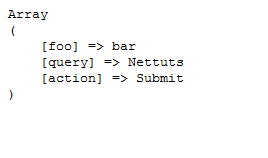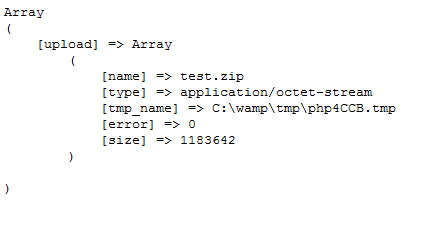# PHP的cURL快速入门

PHP #curl2012-10-31 16:22
cURL 是一个利用URL语法规定来传输文件和数据的工具，支持很多协议，如HTTP、FTP、TELNET等。最爽的是，PHP也支持 cURL 库。本文将介绍 cURL 的一些高级特性，以及在PHP中如何运用它。

```\$content = file_get_contents("http://yige.org");
// or
\$lines = file("http://yige.org");
// or

cURL 是一种功能强大的库，支持很多不同的协议、选项，能提供 URL 请求相关的各种细节信息。

```// 1. 初始化
\$ch = curl_init();
// 2. 设置选项，包括URL
curl_setopt(\$ch, CURLOPT_URL, "http://www.nettuts.com");
curl_setopt(\$ch, CURLOPT_RETURNTRANSFER, 1);
// 3. 执行并获取HTML文档内容
\$output = curl_exec(\$ch);
// 4. 释放curl句柄
curl_close(\$ch);```

```// ...
\$output = curl_exec(\$ch);
if (\$output === FALSE) {
echo "cURL Error: " . curl_error(\$ch);
}
// ...```

```// ...
curl_exec(\$ch);
\$info = curl_getinfo(\$ch);
echo '获取'. \$info['url'] . '耗时'. \$info['total_time'] . '秒';
// ...```

“url” //资源网络地址
“content_type” //内容编码
“http_code” //HTTP状态码
“request_size” //请求的大小
“filetime” //文件创建时间
“ssl_verify_result” //SSL验证结果
“redirect_count” //跳转技术
“total_time” //总耗时
“namelookup_time” //DNS查询耗时
“connect_time” //等待连接耗时
“pretransfer_time” //传输前准备耗时
“starttransfer_time” //开始传输的时间
“redirect_time”//重定向耗时

```// 测试用的URL
\$urls = array(
"http://www.cnn.com",
"http://www.mozilla.com",
);
// 测试用的浏览器信息
\$browsers = array(
"standard" => array (
"user_agent" => "Mozilla/5.0 (Windows; U; Windows NT 6.1; en-US; rv:1.9.1.6) Gecko/20091201 Firefox/3.5.6 (.NET CLR 3.5.30729)",
"language" => "en-us,en;q=0.5"
),
"iphone" => array (
"user_agent" => "Mozilla/5.0 (iPhone; U; CPU like Mac OS X; en) AppleWebKit/420+ (KHTML, like Gecko) Version/3.0 Mobile/1A537a Safari/419.3",
"language" => "en"
),
"french" => array (
"user_agent" => "Mozilla/4.0 (compatible; MSIE 7.0; Windows NT 5.1; GTB6; .NET CLR 2.0.50727)",
"language" => "fr,fr-FR;q=0.5"
)
);
foreach (\$urls as \$url) {
echo "URL: \$url\n";
foreach (\$browsers as \$test_name => \$browser) {
\$ch = curl_init();
// 设置 url
curl_setopt(\$ch, CURLOPT_URL, \$url);
"User-Agent: {\$browser['user_agent']}",
"Accept-Language: {\$browser['language']}"
));
// 页面内容我们并不需要
curl_setopt(\$ch, CURLOPT_NOBODY, 1);
// 返回结果，而不是输出它
curl_setopt(\$ch, CURLOPT_RETURNTRANSFER, 1);
\$output = curl_exec(\$ch);
curl_close(\$ch);
// 有重定向的HTTP头信息吗?
if (preg_match("!Location: (.*)!", \$output, \$matches)) {
echo "\$test_name: redirects to \$matches\n";
} else {
echo "\$test_name: no redirection\n";
}
}
echo "\n\n";
}```http://codeigniter.com/forums/do_search/

`print_r(\$_POST);`

```\$url = "http://localhost/post_output.php";
\$post_data = array (
"foo" => "bar",
"query" => "Nettuts",
"action" => "Submit"
);
\$ch = curl_init();
curl_setopt(\$ch, CURLOPT_URL, \$url);
curl_setopt(\$ch, CURLOPT_RETURNTRANSFER, 1);
// 我们在POST数据哦！
curl_setopt(\$ch, CURLOPT_POST, 1);
// 把post的变量加上
curl_setopt(\$ch, CURLOPT_POSTFIELDS, \$post_data);
\$output = curl_exec(\$ch);
curl_close(\$ch);
echo \$output;````print_r(\$_FILES);`

```\$url = "http://localhost/upload_output.php";
\$post_data = array (
"foo" => "bar",
// 要上传的本地文件地址
);
\$ch = curl_init();
curl_setopt(\$ch, CURLOPT_URL, \$url);
curl_setopt(\$ch, CURLOPT_RETURNTRANSFER, 1);
curl_setopt(\$ch, CURLOPT_POST, 1);
curl_setopt(\$ch, CURLOPT_POSTFIELDS, \$post_data);
\$output = curl_exec(\$ch);
curl_close(\$ch);
echo \$output;```cURL批处理（multi cURL）

cURL还有一个高级特性——批处理句柄（handle）。这一特性允许你同时或异步地打开多个URL连接。

```// 创建两个cURL资源
\$ch1 = curl_init();
\$ch2 = curl_init();
// 指定URL和适当的参数
curl_setopt(\$ch1, CURLOPT_URL, "http://lxr.php.net/");
curl_setopt(\$ch2, CURLOPT_URL, "http://www.php.net/");
// 创建cURL批处理句柄
\$mh = curl_multi_init();
// 加上前面两个资源句柄
// 预定义一个状态变量
\$active = null;
// 执行批处理
do {
\$mrc = curl_multi_exec(\$mh, \$active);
} while (\$mrc == CURLM_CALL_MULTI_PERFORM);
while (\$active && \$mrc == CURLM_OK) {
if (curl_multi_select(\$mh) != -1) {
do {
\$mrc = curl_multi_exec(\$mh, \$active);
} while (\$mrc == CURLM_CALL_MULTI_PERFORM);
}
}
// 关闭各个句柄
curl_multi_remove_handle(\$mh, \$ch1);
curl_multi_remove_handle(\$mh, \$ch2);
curl_multi_close(\$mh);```

WordPress 连接检查器

```// CONFIG
\$db_host = 'localhost';
\$db_user = 'root';
\$db_pass = '';
\$db_name = 'wordpress';
\$excluded_domains = array(
'localhost', 'www.mydomain.com');
\$max_connections = 10;
// 初始化一些变量
\$url_list = array();
\$working_urls = array();
\$not_found_urls = array();
\$active = null;
// 连到 MySQL
if (!mysql_connect(\$db_host, \$db_user, \$db_pass)) {
die('Could not connect: ' . mysql_error());
}
if (!mysql_select_db(\$db_name)) {
die('Could not select db: ' . mysql_error());
}
// 找出所有含有链接的文章
\$q = "SELECT post_content FROM wp_posts
WHERE post_content LIKE '%href=%'
AND post_status = 'publish'
AND post_type = 'post'";
\$r = mysql_query(\$q) or die(mysql_error());
while (\$d = mysql_fetch_assoc(\$r)) {
// 用正则匹配链接
if (preg_match_all("!href=\"(.*?)\"!", \$d['post_content'], \$matches)) {
foreach (\$matches as \$url) {
// exclude some domains
\$tmp = parse_url(\$url);
if (in_array(\$tmp['host'], \$excluded_domains)) {
continue;
}
// store the url
\$url_list []= \$url;
}
}
}
// 移除重复链接
\$url_list = array_values(array_unique(\$url_list));
if (!\$url_list) {
die('No URL to check');
}```

```// 1. 批处理器
\$mh = curl_multi_init();
// 2. 加入需批量处理的URL
for (\$i = 0; \$i < \$max_connections; \$i++) {
}
// 3. 初始处理
do {
\$mrc = curl_multi_exec(\$mh, \$active);
} while (\$mrc == CURLM_CALL_MULTI_PERFORM);
// 4. 主循环
while (\$active && \$mrc == CURLM_OK) {
// 5. 有活动连接
if (curl_multi_select(\$mh) != -1) {
// 6. 干活
do {
\$mrc = curl_multi_exec(\$mh, \$active);
} while (\$mrc == CURLM_CALL_MULTI_PERFORM);
// 7. 有信息否？
// 意味着该连接正常结束
// 8. 从curl句柄获取信息
\$chinfo = curl_getinfo(\$mhinfo['handle']);
// 9. 死链么？
if (!\$chinfo['http_code']) {
// 10. 404了?
} else if (\$chinfo['http_code'] == 404) {
\$not_found_urls []= \$chinfo['url'];
// 11. 还能用
} else {
\$working_urls []= \$chinfo['url'];
}
// 12. 移除句柄
curl_multi_remove_handle(\$mh, \$mhinfo['handle']);
curl_close(\$mhinfo['handle']);
// 13. 加入新URL，干活
do {
\$mrc = curl_multi_exec(\$mh, \$active);
} while (\$mrc == CURLM_CALL_MULTI_PERFORM);
}
}
}
}
// 14. 完了
curl_multi_close(\$mh);
echo "==404 URLs==\n";
echo implode("\n",\$not_found_urls) . "\n\n";
echo "==Working URLs==\n";
echo implode("\n",\$working_urls);
// 15. 向批处理器添加url
static \$index = 0;
// 如果还剩url没用
if (\$url_list[\$index]) {
// 新建curl句柄
\$ch = curl_init();
// 配置url
curl_setopt(\$ch, CURLOPT_URL, \$url_list[\$index]);
// 不想输出返回的内容
curl_setopt(\$ch, CURLOPT_RETURNTRANSFER, 1);
// 重定向到哪儿我们就去哪儿
curl_setopt(\$ch, CURLOPT_FOLLOWLOCATION, 1);
// 不需要内容体，能够节约带宽和时间
curl_setopt(\$ch, CURLOPT_NOBODY, 1);
// 加入到批处理器中
// 拨一下计数器，下次调用该函数就能添加下一个url了
\$index++;
return true;
} else {
// 没有新的URL需要处理了
return false;
}
}```

<img border="0" src="http://nettuts.s3.cdn.plus.org/534_curl/ss_4.png" />

HTTP 认证

```\$url = "http://yige.org/members/";
\$ch = curl_init();
curl_setopt(\$ch, CURLOPT_URL, \$url);
curl_setopt(\$ch, CURLOPT_RETURNTRANSFER, 1);
// 发送用户名和密码
// 你可以允许其重定向
curl_setopt(\$ch, CURLOPT_FOLLOWLOCATION, 1);
// 下面的选项让 cURL 在重定向后
// 也能发送用户名和密码
curl_setopt(\$ch, CURLOPT_UNRESTRICTED_AUTH, 1);
\$output = curl_exec(\$ch);
curl_close(\$ch);```

FTP 上传

PHP 自带有 FTP 类库， 但你也能用 cURL：

```// 开一个文件指针
\$file = fopen("/path/to/file", "r");
// url里包含了大部分所需信息
\$ch = curl_init();
curl_setopt(\$ch, CURLOPT_URL, \$url);
curl_setopt(\$ch, CURLOPT_RETURNTRANSFER, 1);
// 上传相关的选项
curl_setopt(\$ch, CURLOPT_INFILE, \$fp);
curl_setopt(\$ch, CURLOPT_INFILESIZE, filesize("/path/to/file"));
// 是否开启ASCII模式 (上传文本文件时有用)
curl_setopt(\$ch, CURLOPT_FTPASCII, 1);
\$output = curl_exec(\$ch);
curl_close(\$ch);```

```\$ch = curl_init();
curl_setopt(\$ch, CURLOPT_URL,'http://yige.org');
curl_setopt(\$ch, CURLOPT_RETURNTRANSFER, 1);
// 指定代理地址
curl_setopt(\$ch, CURLOPT_PROXY, '11.11.11.11:8080');
// 如果需要的话，提供用户名和密码
curl_setopt(\$ch, CURLOPT_PROXYUSERPWD,'user:pass');
\$output = curl_exec(\$ch);
curl_close (\$ch);```

```\$ch = curl_init();
curl_setopt(\$ch, CURLOPT_URL,'http://yige.org');
curl_setopt(\$ch, CURLOPT_WRITEFUNCTION,"progress_function");
curl_exec(\$ch);
curl_close (\$ch);
function progress_function(\$ch,\$str) {
echo \$str;
return strlen(\$str);
}```